The road to acids

• The definition of acids and bases have changed over time.
• At AS we looked at the definition of acid base behaviour as defined by Bronsted - Lowrey in 1923.
• They defined:

Acids - proton donors

Base - proton acceptors

Alkali - a base that dissolves in water forming OH- ions

Qu 1-2  P135

The role of H+ in reactions of acids

• A hydrogen ion is just a hydrogen atom that has lost an electron.

• As hydrogen only has 1 electron this means that a hydrogen ion is just a proton.

• H+ and proton mean the same.

• In water the acid dissociates producing hydrogen ions / protons:

 HCl(g) + aq g H+(aq) + Cl-(aq)
 H2SO4(l) + aq g H+(aq) + HSO4-(aq)

Mono, di and tri - basic acids:

• Acids are categorised on the number of acidic hydrogen's / protons they can release:

Monobasic: releases 1 proton

 HCl(aq) + aq g H+(aq) + Cl-(aq)

Dibasic: releases 2 protons

 H2SO4(aq) + aq g H+(aq) + HSO4-(aq)
 HSO4-(aq) + aq D H+(aq) + SO42-(aq)

Tribasic: releases 3 protons

 H3PO4(aq) + aq D H+(aq) + H2PO4-(aq)
 H2PO4-(aq) + aq D H+(aq) + HPO42-(aq)
 HPO4-(aq) + aq D H+(aq) + PO43-(aq)

Acid - base reactions:

• Acids react with:

Carbonates

Bases (metal oxides)

Alkalis (metal hydroxides)

• In every reaction the acid is neutralised and water is formed as part of the reaction.

• Looking at the ionic equation we can see why water is formed in each case.

• This means we have to remove the spectator ions

• These are species that do not change from reactants to products (including the state symbols).

• It doesn't matter whether the acid is strong or weak as acids will release more H+ ions as they are used up in the reaction LCP

Carbonates:

 Full: 2HCl(aq) + CaCO3(s) g CaCl2(aq) + CO2(g) + H2O(l) Ions: 2H+(aq) + 2Cl-(aq) + CaCO3(s) g Ca2+(aq) + 2Cl-(aq) + CO2(g) + H2O(l) Ionic: 2H+(aq) + CaCO3(s) g Ca2+(aq) + CO2(g) + H2O(l)
• If the carbonate is in solution it simplifies further:

 Full: 2HCl(aq) + CaCO3(aq) g CaCl2(aq) + CO2(g) + H2O(l) Ions: 2H+(aq) + 2Cl-(aq) + Ca2+(aq) + CO32-(aq) g Ca2+(aq) + 2Cl-(aq) + CO2(g) + H2O(l) Ionic: 2H+(aq) + CO32-(aq) g + CO2(g) + H2O(l)

Bases:

 Full: 2HNO3(aq) + MgO(s) g Mg(NO3)2(aq) + H2O(l) Ions: 2H+(aq) + 2NO3-(aq) + MgO(s) g Mg2+(aq) + 2NO3-(aq) + H2O(l) Ionic: 2H+(aq) + MgO(s) g Mg2+(aq) + H2O(l)

Alkalis:

 Full: H2SO4(aq) + 2KOH(aq) g K2SO4(aq) + 2H2O(l) Ions: 2H+(aq) + SO42-(aq) + 2K+(aq) + 2OH-(aq) g 2K+(aq) + SO42-(aq) + 2H2O(l) Ionic: 2H+(aq) + 2OH-(aq) g 2H2O(l)

Redox reactions of acids with metals:

• These are not acid base reactions as water is not made, they are just REDOX reactions:

 Full: 2HCl(aq) + Mg(s) g MgCl2(aq) + H2(g) Ions: 2H+(aq) + 2Cl-(aq) + Mg(s) g Mg2+(aq) + 2Cl-(aq) + H2(g) Ionic: 2H+(aq) + Mg(s) g Mg2+(aq) + H2(g)
• These type of reactions are acids behaving as strong oxidising agents.
• Conc. nitric and sulphuric acids are examples of these types of reactions.

Qu 1 - 2  P137

Conjugate acid - base pairs

Acids as proton donors:

• Acids are molecules that contain hydrogen that can be released as H+
• This will only donate protons if there is something to accept it - a base

Hydronium ions:

• Aqueous acids donate their protons to water.  This makes water a proton acceptor forming the hydronium ion / oxonium ion:

 HCl(aq) + H2O(l) g H3O+(aq) + Cl-(aq)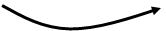hydronium ion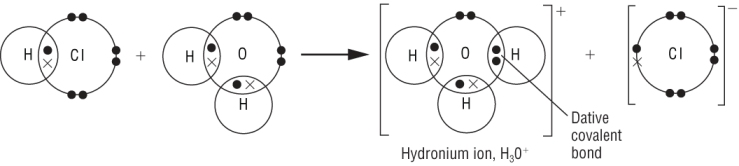• This reaction is often simplified to:
 HCl(aq) g H+(aq) + Cl-(aq) hydronium ion
• H+(aq) is a simplified way of writing H3O+(aq)

Acid - base pairs: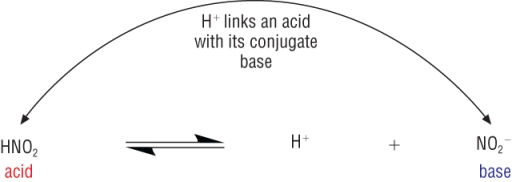• The forward reaction is when a proton is donated to water forming ions.

• Strong acids fully dissociate meaning products predominate.

• Now consider the reverse reaction.

• The proton is now accepted by the NO2-.

• This makes this an acid - base pair

Conjugate pairs

• There is a relationship between 2 of the species, one on either side of the equilibrium and they can be considered conjugate pairs:

 Conjugate pair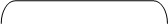HNO2(aq) + H2O(l) D H3O+(aq) + NO2-(aq) acid base acid base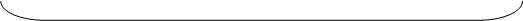Congugate pair
• Look at the NO2 species on both side of the equilibrium.  They are in the acid and the base (an acid / base pairpairs.

• Another Conjugate acid / base pair can be seen in the following reaction:

 Conjugate pairNH3(aq) + H2O(l) D OH-(aq) + NH4+(aq) base acid base acidCongugate pair

Qu 1 - 2  P139

What is pH?

The pH scale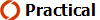• Aqueous acids contain [H+] in the range from 101 10 10-15 Mol dm-3
• To compress such a large scale we use a negative logarithmic scale / - logs
• This reduces the scale to whole numbers and deals with the small concentrations of [H+]
• This is the origin of the pH scale which gives us a relationship between pH and the hydrogen concentration:

 pH  =  -log10[H+(aq)]
• OR

 [H+(aq)]  =  10-pH

What does pH mean:

 Low pH High [H+(aq)] High pH Low [H+(aq)]

Converting between pH and [H+(aq)]

• [H+(aq)] and pH are not usually whole numbers and may not be in standard form.
• To calculate the pH you will need your calculator: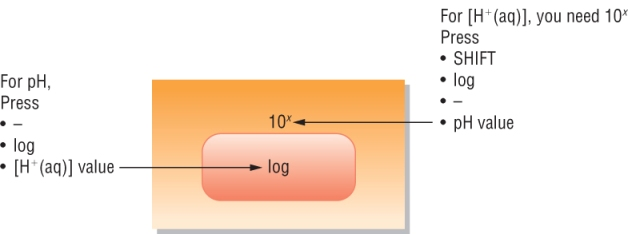Calculating the pH of strong acids:

## Weak acids – do no donate protons to water very readily (later)

• Consider the following:
 Water HCl(g) g H+(aq) + Cl-(aq)
 1 mole 1 mole 1 mole
• This means that for strong acids the [H+(aq)]  =  [ACID]

Examples:

1)  Calculate the pH of 1 x 10-3 mol dm3 HCl:

[HCl(aq)] = 1 x 10-3 mol dm3

[H+(aq)] = 1 x 10-3 mol dm3

pH  =  - log [H+(aq)]

pH  =  - log [1 x 10-3]

pH  =  3

2)  Calculate the pH of 1 x 10-6 mol dm-3 HNO3

[HCl(aq)] = 1 x 10-6 mol dm3

[H+(aq)] = 1 x 10-6 mol dm3

pH  =  - log [H+(aq)]

pH  =  - log [1 x 10-6]

pH  =  6

Qu  1 - 3  P141

The ionisation of water

The ionisation of water and Kw

• We always assume that water does not act as an acid or base in reactions.

• Water plays a big part in the understanding of acids.

• In order to understand acids then we need to understand water first:

Water: facts and models

Water conducts electricity.  It has a conductivity of 26.6 x 10-6 Scm-1

• The fact that water conducts at all means that there must be at least some ions present:

 H2O(l) D H+(aq) + OH-(aq)
• This means that water would have an equilibrium expression:
 Kc = [H+]  x  [OH-] [H2O]
• Rearrange this to:
 Kc   x   [H2O] = [H+]  x  [OH-]
• [H2O] of water in water is always constant.
• Kc is also a constant.  These 2 terms can be replaced with one constant which we call
• Kw is the ionic product of water (basically an kind of equilibrium constant just for water):
 Kc   x   [H2O] = [H+]  x  [OH-]
 Kw = [H+]  x  [OH-]

Kw has an equilibrium constant of 1 x 10-14

PH and the ionic product of water

 Kw = [H+]  x  [OH-]
• Where Kw is known as the ionic product of water.
• At 298K the ionic product of water is 1 x 10-14 Mol2 dm-6.
 Kw = [H+]  x  [OH-] = 1 x 10-14
• The protons and hydroxides are both produced from the dissociation of water.

• This means that the concentrations of both of these ions must be the same (as they both came from water).

 [H+] = [OH-]
• This means that in pure water at equilibrium:

 [H+] x [OH-] = 1 x 10-14 [H+]2 = 1 x 10-14 [H+] = (1 x 10-14)0.5 where ( )0.5 = square root [H+] = 1 x 10-7
• The concentration of each of the ions produced by the dissociation of water = 1 x 10-7 Mol dm-3
• We know that the pH of water = 7
• A negative logarithmic scale compresses the values into a smaller range and gives us positive values, more useful to use as comparisons.

• This is the origin of the pH scale which gives us a relationship between pH and the hydrogen concentration:

 pH  =  -log10[H+(aq)]

The significance of Kw:

• Kw is basically an equilibrium expression linking [H+] and [OH-].

• We have already seen that at the pH of water = 7 when the [H+] = [OH-].

 Kw = [H+]  x  [OH-]
 Acidic conditions [H+] > [OH-]. Kw must always equal 1 x 10-14 Alkaline conditions [H+] < [OH-]. [H+]  x  [OH-]  =  1 x 10-14

The link between [H+] and [OH-]

• The [H+] and [OH-].are linked by the ionic product of water.

 Kw = [H+]  x  [OH-] = 1 x 10-14
• A few examples:
 pH 1 3 5 7 9 11 13 [H+] 10-1 10-3 10-5 10-7 10-9 10-11 10-13 [OH-] 10-13 10-11 10-9 10-7 10-5 10-3 10-1
• This means it is possible to calculate [H+] and hence pH from [OH-]

Qu  1 - 3  P147

pH of strong bases

## Weak bases – do not dissociate completely to generate OH- ions in water (later)

• Consider the following:
 Water NaOH(s) g Na+(aq) + OH-(aq)
 1 mole 1 mole 1 mole
• We use the ionic product of water to calculate the corresponding [H+] concentration for bases:

 Kw = [H+]  x  [OH-] = 1 x 10-14

Example:  0.1M solution of NaOH

 [H+] x [OH-] = 1 x 10-14 [H+] x 0.1 = 1 x 10-14 [H+] = 1 x 10-14 0.1 [H+] = 1 x 10-13 pH = -log10[H+(aq)] pH = -log10[1 x 10-13] pH = 13

There is a quicker way:

• If you take -log10 [OH-] (essentially a 'pOH') you can use math's of indices to calculate the pH bases on the ionic product of water, Kw.
• If you take -log10 of the ionic product of water, Kw:
 [H+] x [OH-] = 1 x 10-14 -log10 [H+] x -log10 [OH-] = -log10 (1 x 10-14) pH + pOH = 14

Qu  1 - 3  P149

Acid - base equilibria:

• Remember, in aqueous solutions acids dissociate.

Strong acids:

• They dissociate fully in water (100%) if they are strong acids:
 Water HA(aq) g H+(aq) + Cl-(aq)

Weak acids:

• They partially dissociate in water if they are weak acids:
 Water HA(aq) D H+(aq) + Cl-(aq)
 1 mole 0 moles 0 moles
 0.99 moles 0.01 moles 0.01 moles
• This gives us an equilibrium, using ethanoic acid as an example:
 Water
 CH3COOH(l) D H+(aq) + CH3COO-(aq)
 0.99 moles 0.01 moles 0.01 moles
• 1 mole of ethanoic acid dissociates to 0.99 moles of ethanoic acid forming 0.01 mole of each of the ions.
• The equilibrium lies well over to the LHS
• This means an equilibrium expression can be written:

The acid dissociation constant, Ka

• For ethanoic acid:
 CH3COOH(l) D H+(aq) + CH3COO-(aq)
• An equilibrium expression can be written:
 Ka = [H+]  x  [CH3COO-] [CH3COOH]
• Notice that we used Kc for concentrations.  We use Ka when dealing with acids.
• Ka is known as the acid dissociation constant and this can be either calculated or looked up in a table for use in calculations.
• Ethanoic acid has a Ka = 1.7 x 10-5 Mol dm-3.

Units of Ka

• These are worked out in exactly the same way as for Kc:
 Ka = [H+]  x  [CH3COO-] [CH3COOH] Ka = mol dm-3  x  mol dm-3 mol dm-3 Ka = mol dm-3  x  mol dm-3 mol dm-3 Ka = mol dm-3 Large Ka Large dissociation - Strong acid Small Ka Small dissociation - Weak acid

Ka and pKa - a compressed scale:

 Ka = [H+]  x  [A-] [HA]
• Just like the pH scale it is more useful to compress the scale.
• To keep it simple we use the same logarithmic scale when dealing with Ka values.
• Now, instead of pH we have pKa :
 pKa  =  -log10[Ka]
• If it is a strong acid then it will dissociate a lot.  This gives a large top number and a small bottom number.
• This will give a large Ka value, but a small pKa
• If it is a weak acid then it will dissociate a little.  This gives a small value on the top and a large bottom number.
• This will give a small Ka value, but a large pKa

Calculating the pH for weak acids

• The pH of a weak acid is more difficult to calculate as the acid does not completely dissociate.
 HA(aq) D H+(aq) + A-(aq) 1M 0M 0M 0.99M 0.01M 0.01M
• The [H+(aq)] is not the same as the [HA(aq)]
• It is calculated in 2 steps:

Step 1:  [H+(aq)] must be calculated from the acid equilibrium expression, Ka:

 Ka = [H+]  x  [A-] [HA]
• In order to calculate the [H+(aq)] we have to make a couple of assumptions:  LEARN

1)    For a weak acid:    [H+(aq)]    =    [A-(aq)]    - as both have been formed from the dissociation of the acid.

2)    [HA(aq)] remains unchanged - a weak acid dissociates so little that the concentration remains virtually the same.

 Ka = [H+]2 [HA]
• Rearrange to get:
 [H+]2 = Ka  x  [HA]
 [H+] = (Ka  x  [HA])0.5

Step 2:  [H+(aq)] is put in the pH formula:  pH  =  -log10[H+(aq)]

Example:  Calculate the pH of a 0.100 mol dm-3 ethanoic acid, Ka = 1.7 x 10-5 mol dm-3

• Write the equation for the dissociation of the acid:
 CH3COOH(l) D H+(aq) + CH3COO-(aq)

Step 1:  [H+(aq)] must be calculated from the acid equilibrium expression, Ka:

 Ka = [H+]  x  [CH3COO-] [CH3COOH]
• Apply the assumptions:

1)    [H+(aq)]    =    [CH3COO-]

2)    [CH3COOH] remains unchanged

 Ka = [H+]2 [CH3COOH]
• Put in the numbers:
 1.7 x 10-5 = [H+]2 0.100
• Rearrange:
 [H+]2 = 1.7 x 10-5  x  0.100
• Take the square root:
 [H+] = 1.304  x  10-3 mol dm-3
• Now put the [H+(aq)] in the pH formula:
 pH =  -log10[H+(aq)]
 pH =  -log10 [1.3  x  10-3]
 pH =  2.88

Summary:

Step 1:  Calculate [H+(aq)]

 [H+] = (Ka  x  [HA])0.5

Applying assumptions:

1)    [H+(aq)]    =    [A-(aq)]

2)    [HA(aq)] remains unchanged

Step 2:  Calculate pH

pH  =  -log10[H+(aq)]

Calculating Ka for weak acids

• This can of course work the other way to calculate the Ka of a weak acid
• For this we need:

1)    pH of the weak acid

2)    Concentration of the weak acid

• Again is is done in 2 steps:

Step 1:  Calculate [H+(aq)]

• This is done from the pH and working backwards:
 [H+] = 10 - pH

Step 2:  Calculate Ka

• Put the [H+] and [HA] to calculate Ka
 Ka = [H+]2 [HA]

Example - Calculate Ka for 0.03 mol dm-3 methanoic acid, pH = 2.66

Step 1:  Calculate [H+(aq)]

 [H+] = 10 - pH
 [H+] = 10 - 2.66
 [H+] = 2.19  x  10-3

Step 2:  Calculate Ka

• Put the [H+] and [HA] to calculate Ka
 Ka = [H+]2 [HA]
• Put values in:
 Ka = [2.19  x  10-3]2 [0.03]
 Ka = 1.6  x  10-4 Mol dm-3

Qu 1 - 3  P 143  /  Qu 1 - 2  P145

Buffer solutionsA buffer solution minimises the change in pH with the small addition of acids or bases
• These are important in controlling the pH in living systems.
• Buffer solutions are made:

1)  Weak acid, HA

2)  Conjugate base of the weak acid, A-

• Ethanoic acid is a weak acid being only slightly ionised in solution

• The equilibrium lies over to the left:

 CH3COOH(aq) D H+(aq) + CH3COO-(aq)
• What would happen if more ethanoate ions were added to the solution.

• This can be done by adding a small amount of sodium ethanoate which is highly ionised in solution:

 CH3COO-  Na+(aq) g Na+(aq) + CH3COO-(aq)

Alternativily:

• Buffer solutions can also be made by partially neutralising an acid:
 HCOOH(aq) + NaOH(aq) g HCOO-  Na+(aq) + H2O
• This leaves some of the acid to dissociate partially:
 HCOOH(aq) D H+(aq) + HCOO-(aq)
• It also produces the ionic salt which dissociates fully in solution:
 HCOO-  Na+(aq) g Na+(aq) + HCOO-(aq)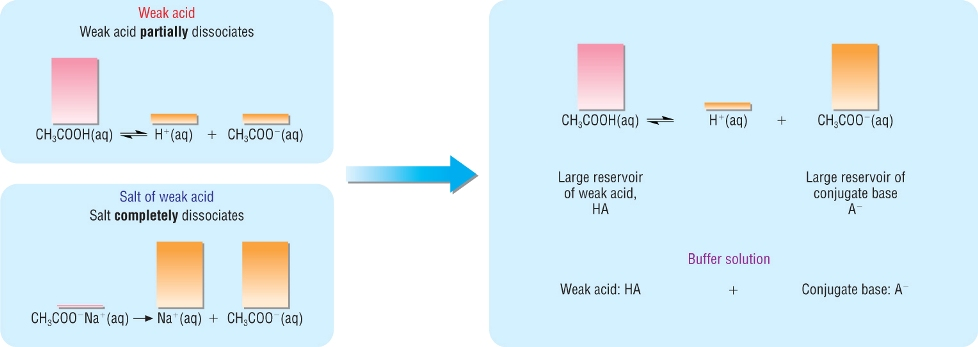• This gives you an equilibrium with a large amount of acid and the conjugate base.
• The high concentration of the conjugate base pushes the acid equilibrium over to the LHS.
• This means that the [H+(aq)] will be very small.

How does a buffer act?

• The 2 reactions needed to explain how they work are outlined below:
 HA(aq) D H+(aq) + A-(aq) Reaction 1
 H+(aq) + OH-(aq) g H2O(l) Reaction 2

Addition of and acid, H+ ions:

• Addition of H+ ions increases the [H+]
• This moves the equilibrium of Reaction 1 to the left hand side
• This reduces the [H+] thus restoring the pH.

Addition of an alkali, OH- ions:

• OH- ions reacts with H+ ions in Reaction 1 according to Reaction 2 forming water.
• This reduces the [H+] in Reaction 1.
• The equilibrium in Reaction 1 moves to the right hand side replacing the H+ used, thus restoring the pH

Overall: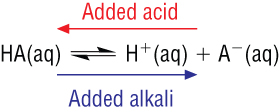Qu 1,2  P151

pH values of buffer solutions

Calculations involving buffer solutions

• We can calculate the pH of a buffer solution if we are given:

1)    Ka of the weak acid

2)    Equilibrium concentrations of the conjugate acid - base pair

Method 1:

1)    Write the reaction:

 HA(aq) D H+(aq) + A-(aq)

2)    Write the equilibrium expression, Ka

 Ka = [H+]  x  [A-] [HA]
• Remember [H+]  does not equal  [A-] as [A-] is determined from the conjugate base

3)    Rearrange the equation to calculate the hydrogen concentration

 [H+] = Ka    x [HA] [A-]
• 2 assumptions are made here

1)  [HA] remains unchanged as it dissociates by such a small amount

2)  [A-]  =  [NaA]  as is dissociates fully

4)    calculate the pH

 pH =  -log10[H+(aq)]

Example:

• A buffer solution contains 0.600 Mol dm-3 propanoic acid and 0.800 Mol dm-3 sodium propanoate.
• The equilibrium constant, Ka for propanoic acid = 1.3  x  10-5 Mol dm-3.
• Calculate the pH of the buffer solution:

1)    Write the reaction:

 C2H5COOH(aq) D H+(aq) + C2H5COO-(aq)

2)    Write the equilibrium expression, Ka

 Ka = [H+]  x  [C2H5COO-] [C2H5COOH]

3)    Rearrange the equation to get the hydrogen ion concentration:

 [H+] = Ka    x [C2H5COOH] [C2H5COO-]

 [H+] = 1.3  x  10-5    x 0.600 0.800

 [H+] = 9.75  x  10-6

6)    calculate the pH

 pH =  -log10[H+(aq)]
 pH =  -log10 [9.75  x  10-6]
 pH =  5.01

Method 2:

• Use theHenderson - Hasselbalch relationship: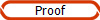pH = pKa +    log [A-] [HA]

 pH = - logKa +    log [C2H5COO-] [C2H5COOH]

 pH = - log  1.3  x  10-5 +    log [0.800] [0.600]

 pH = 5.01

The carbonic acid - hydrogen carbonate buffer system

• The pH of blood needs to be between 7.35 - 7.45.
• This is controlled by a mixture of buffers but the carbonic acid buffer is the most important:

Carbonic acid:    H2CO3                   and its conjugate base:    HCO3-

• The 2 reactions needed to explain how this buffer works is outlined below:
 H2CO3(aq) D H+(aq) + HCO3-(aq) Reaction 1
 H+(aq) + OH-(aq) g H2O(l) Reaction 2

Addition of and acid, H+ ions:

• Addition of H+ ions increases the [H+]
• This moves the equilibrium of Reaction 1 to the left hand side
• This reduces the [H+] thus restoring the pH.

Addition of an alkali, OH- ions:

• OH- ions reacts with H+ ions in Reaction 1 according to Reaction 2 forming water.
• This reduces the [H+] in Reaction 1.
• The equilibrium in Reaction 1 moves to the right hand side replacing the H+ used, thus restoring the pH

Overall: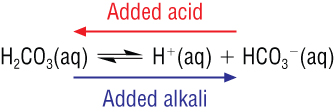• Most materials released into the blood is acidic.
• This is removed by the conversion into carbonic acid.
• Enzymes convert the carbonic acid into dissolved CO2 which is released as a gas through the lungs:
 CO2(aq) D CO2(g)
• The amount of CO2 is controlled by breathing.  Breathing heavily removes more CO2 from the blood.Qu  1,2  P153

Neutralisation - titration curvesTitrations for AS chemistry

• During titrations you are measuring the exact volume of one solution that reacts with a known volume of another.
• This is called the equivalence point.
• Acid - base titrations deals with the pH changes that occur during the movement from acid g base and from base g acid

Key features of titration curves - for a base added to an acid: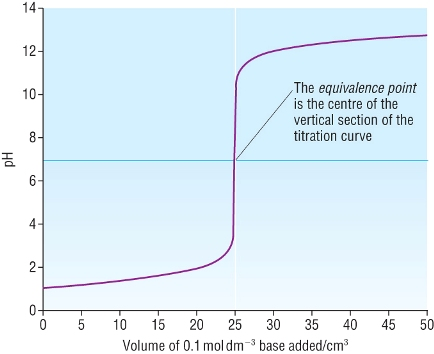When the base is first added, the pH increases very slightly due to the large excess of acid, the gradually increases. Within a drop, the pH increases sharply from below 7 to above 7, the middle of this section is the equivalence point. With continual addition of base, the increase in pH tails of until the increases is again very slight.

Choosing the indicator

• Many dyes are affected by pH as they are themselves weak acids.
• This means that they will themselves be subject to shifts in their equilibrium with the addition of H+ or OH-.
• This will then correspond to a colour change.
• They are often considered to be weak acids where the acid or corresponding base are both coloured.
• Consider an indicator, HIn
 HIn(aq) D H+(aq) + In-(aq)
• Addition of an acid will move the equilibrium to the reactants.  This shifts to the RED

• Addition of a base removes H+(aq) which moves the equilibrium to the products.  This shifts to the YELLOW

• When the [HIn]  =  [In-] the indicator is at its end point

• Most indicators change colour over a couple of pH units.

• The end point for chemical indicators is in the middle of the pH range.

Indicator and titration curvesThe equivalence point of any neutralisation reaction is pH7 The end point (colour change) in indicators do not necessarily correspond to the equivalence point Look at methyl orange - it changes colour in the pH range of 2.8 - 4.0. This means that the end point does not necessarily correspond to the equivalence point for some neutralisation reactions
• Acids and alkalis can be strong or weak which gives us 4 types of neutralisation: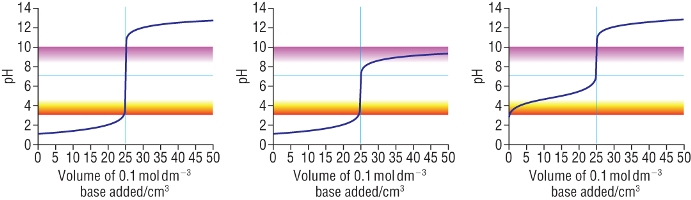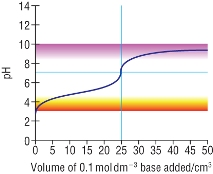Strong acid – Strong base Strong acid – Weak base Weak acid – Strong base Weak acid – Weak base Phenylphthalein - Suitable Phenylphthalein - Unsuitable Phenylphthalein - Suitable Phenylphthalein - Unsuitable Methyl orange - Suitable Methyl orange - Suitable Methyl orange - Unsuitable Methyl orange - Unsuitable
• Weak acids - weak alkalis are not titrated against each other due to the short pH change during neutralisationQu 1-2  P155

Neutralisation - enthalpy changes

Standard enthalpy change of neutralisation

• Consider the neutralisation of aqueous acid with aqueous base:

 Full: HCl(aq) + NaOH(aq) g NaCl(aq) + H2O(l) Ions: H+(aq) + Cl-(aq) + Na+(aq) + OH-(aq) g Na+(aq) + Cl-(aq) + H2O(l) Ionic: H+(aq) + OH-(aq) g H2O(l)
• Na+(aq)  and Cl-(aq) are spectator ions and do not take part in the reaction.

• The enthalpy change for this is the enthalpy change of neutralisation:

 Is the energy change when aq acid is neutralised by aq base forming 1 mole of water under standard conditions

Determination of enthalpy change of neutralisation:

Example:

25cm3 of 2M nitric acid is added to 25cm3 of 2M potassium hydroxide.  The temperature increases from 22.0 oC to 35.5 oC.

Calculate the enthalpy change of neutralisation:

1)  The energy change:

 Q = m x c x DT Q = 50 x 4.18 x 13.5 Q = 50 x 4.18 x 13.5 Q = 2821.5 Joules Exothermic so will be negative: Q = - 2821.5 Joules

2)  Calculate the number of moles used:

 Moles (HNO3) = C x V 1000 Moles (HNO3) = 2 x 25 1000 Moles (HNO3) 0.0500
 Moles (KOH) = C x V 1000 Moles (KOH) = 2 x 25 1000 Moles (KOH) 0.0500

3)  Calculate the amount of energy exchanged per mole of water, enthalpy change of neutralisation:

Enthalpy = Energy

Moles

Enthalpy =  - 2821.5

0.0500

Enthalpy = - 56430 j Mol-1

Enthalpy = - 56.4 Kj Mol-1

• The number of moles of acid : alkalil : water are the same for monobasic acids

Comparisons of enthalpy changes of neutralisation of different acids:

Acid

Reaction

DHqneut  /  Kj Mol-1

Hydrochloric acid
 Full: HCl(aq) + NaOH(aq) g NaCl(aq) + H2O(l)
 Ionic: H+(aq) + OH-(aq) g H2O(l)
- 57.9
Nitric acid
 Full: HNO3(aq) + NaOH(aq) g NaNO3(aq) + H2O(l)
 Ionic: H+(aq) + OH-(aq) g H2O(l)
- 57.6
Hydrobromic acid
 Full: HBr(aq) + NaOH(aq) g NaBr(aq) + H2O(l)
 Ionic: H+(aq) + OH-(aq) g H2O(l)
- 57.6
Ethanoic acid
 Full: CH3COOH(aq) + NaOH(aq) g CH3COONa(aq) + H2O(l)
 Ionic: H+(aq) + OH-(aq) g H2O(l)
- 57.1
• Note that they are all around the same enthalpy changes.
• This is due to the fact that it is essentially the same ionic reaction once the spectator ions have been removed
• Ethanoic acid has a slightly lower enthalpy change due to the fact that ethanoic acid has to dissociate as the reaction proceeds.
• This means that the reaction is slower so energy is lost as it proceeds at a slower rate.Qu 1 - 2  P157  /  Qu 6 - 9  P159  /  5 - 9  P163